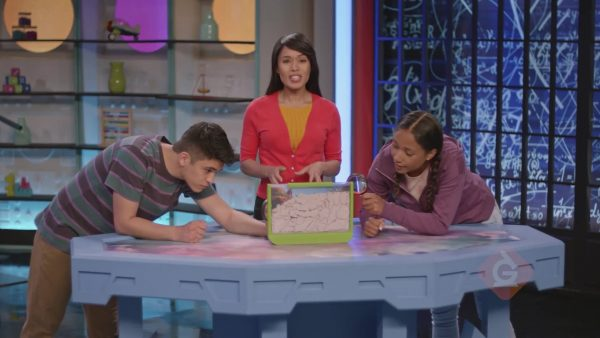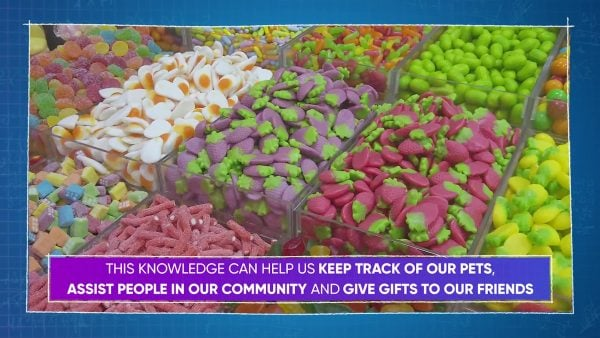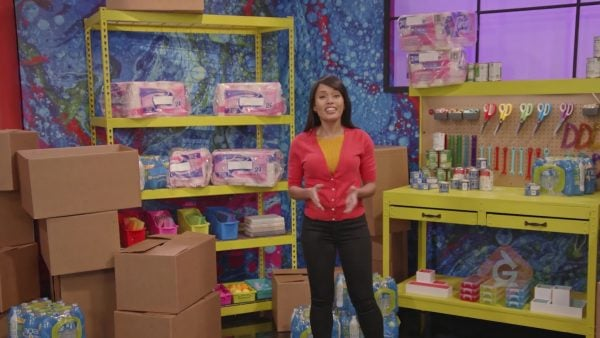Solve 2 Step Problems with 4 Operations | for kids 3rd, 4th, and 5th Grade
1%
It was processed successfully!WHAT IS SOLVING 2 STEP PROBLEMS WITH 4 OPERATIONS?

Draw on your knowledge of addition, subtraction, multiplication, and division, and learn to identify keywords in a word problem to decide on the necessary operations and the order in which you must be followed in order to find the solution.

To better understand solving 2 Step Problems with 4 Operations…

WHAT IS SOLVING 2 STEP PROBLEMS WITH 4 OPERATIONS?. Draw on your knowledge of addition, subtraction, multiplication, and division, and learn to identify keywords in a word problem to decide on the necessary operations and the order in which you must be followed in order to find the solution. To better understand solving 2 Step Problems with 4 Operations…

## LET’S BREAK IT DOWN!

### AntsConsider a word problem: Marcos and April have an ant farm. They start with 27 ants. Then they get 68 more ants. Finally, they give away 23 ants. How many ants are left in April and Marcos's farm? Which words give us clues about the operations we need to use? “More” suggests addition, and “give away” suggests subtraction. So, they start with 27 ants, add 68 ants, and subtract 23 ants. If we call the number of ants at the end ‘a’, we can write the equation 27 + 68 – 23 = a to represent this situation. First, perform the addition. 27 + 68 = 95. Then subtract, 95 – 23 = 72. Therefore, the number of ants left is equal to 72. Or, a = 72. Try this one yourself: June’s bookstore has 853 books. On Monday, 326 books are sold. At the end of the day, 472 new books are delivered to the store. How many books does June have now?

Ants Consider a word problem: Marcos and April have an ant farm. They start with 27 ants. Then they get 68 more ants. Finally, they give away 23 ants. How many ants are left in April and Marcos's farm? Which words give us clues about the operations we need to use? “More” suggests addition, and “give away” suggests subtraction. So, they start with 27 ants, add 68 ants, and subtract 23 ants. If we call the number of ants at the end ‘a’, we can write the equation 27 + 68 – 23 = a to represent this situation. First, perform the addition. 27 + 68 = 95. Then subtract, 95 – 23 = 72. Therefore, the number of ants left is equal to 72. Or, a = 72. Try this one yourself: June’s bookstore has 853 books. On Monday, 326 books are sold. At the end of the day, 472 new books are delivered to the store. How many books does June have now?

### Candy StoreNow look at a problem using addition and multiplication: You and your friend Jasmine are buying candy at a candy store. Jasmine buys 4 packs with 8 pieces of candy in each pack. You buy 5 packs with 6 pieces in each pack. How many pieces of candy did you buy all together? To restate, we need to multiply to find out how many pieces of candy Jasmine has, and we also need to multiply to find out how many pieces you have. Then we need to add to find the combined number of pieces of candy. Jasmine has 4 × 8 = 32 pieces of candy, and you have 5 × 6 = 30 pieces. Together, you have 32 + 30 = 62 pieces of candy. Try this one yourself: At a birthday party, 12 kids eat 2 slices of pizza each, and 5 adults eat 3 slices each. How many slices of pizza were eaten altogether?

Candy Store Now look at a problem using addition and multiplication: You and your friend Jasmine are buying candy at a candy store. Jasmine buys 4 packs with 8 pieces of candy in each pack. You buy 5 packs with 6 pieces in each pack. How many pieces of candy did you buy all together? To restate, we need to multiply to find out how many pieces of candy Jasmine has, and we also need to multiply to find out how many pieces you have. Then we need to add to find the combined number of pieces of candy. Jasmine has 4 × 8 = 32 pieces of candy, and you have 5 × 6 = 30 pieces. Together, you have 32 + 30 = 62 pieces of candy. Try this one yourself: At a birthday party, 12 kids eat 2 slices of pizza each, and 5 adults eat 3 slices each. How many slices of pizza were eaten altogether?

### DonationsAdesina, April, and Marcos make 6 care packages a day for 4 days, then they split those packages up between 3 community centers. How many packages does each community center get? To restate the problem, we first need to multiply to find out how many packages they made in all, and then we need to divide to find out how many packages each community center receives. Let c stand for the number of care packages each community center receives. For the first step, we multiply: 4 × 6 = 24, so they made 24 packages in all. 24 ÷ 3 = 8, so each community center receives 8 packages. Then 24 ÷ 3 = c, and c = 8. Try this one yourself: For 5 days, you earn \$35 a day helping your neighbors with their yard. You then use all of your money to buy 25 identical stuffed toys to donate to a local shelter. How much does each toy cost?

Donations Adesina, April, and Marcos make 6 care packages a day for 4 days, then they split those packages up between 3 community centers. How many packages does each community center get? To restate the problem, we first need to multiply to find out how many packages they made in all, and then we need to divide to find out how many packages each community center receives. Let c stand for the number of care packages each community center receives. For the first step, we multiply: 4 × 6 = 24, so they made 24 packages in all. 24 ÷ 3 = 8, so each community center receives 8 packages. Then 24 ÷ 3 = c, and c = 8. Try this one yourself: For 5 days, you earn \$35 a day helping your neighbors with their yard. You then use all of your money to buy 25 identical stuffed toys to donate to a local shelter. How much does each toy cost?

### Bouncy BallsApril buys 123 bouncy balls. She keeps 42 for herself and divides the rest evenly among her 9 friends. How many bouncy balls does each friend get? To restate the question, start with 123 balls, subtract 42 balls, and divide the rest of the balls into 9 equal groups. Call the number of balls each friend gets b. The first step is subtraction: 123 – 42 = 81. The second step is division: 81 ÷ 9 = 9. Then 81 ÷ 9 = b, so b = 9. Each friend gets 9 bouncy balls. Try this one yourself: Tyler is on vacation for 60 days. He wants to spend the first 11 days watching movies, and the remainder of the time reading books. If it takes 7 days to read a book, how many books can he read on vacation?

Bouncy Balls April buys 123 bouncy balls. She keeps 42 for herself and divides the rest evenly among her 9 friends. How many bouncy balls does each friend get? To restate the question, start with 123 balls, subtract 42 balls, and divide the rest of the balls into 9 equal groups. Call the number of balls each friend gets b. The first step is subtraction: 123 – 42 = 81. The second step is division: 81 ÷ 9 = 9. Then 81 ÷ 9 = b, so b = 9. Each friend gets 9 bouncy balls. Try this one yourself: Tyler is on vacation for 60 days. He wants to spend the first 11 days watching movies, and the remainder of the time reading books. If it takes 7 days to read a book, how many books can he read on vacation?

## SOLVE 2 STEP PROBLEMS WITH 4 OPERATIONS VOCABULARY

Operations
Putting two numbers together to find their total.
Subtraction
Taking away a number from another number.
Multiplication
Division
Splitting a quantity into equal groups.
Restate
To say again in your own words.
Stating that one expression is equal to another.

## SOLVE 2 STEP PROBLEMS WITH 4 OPERATIONS DISCUSSION QUESTIONS

### Why is it important to restate the problem in your own words?

You should restate the problem in your own words to make sure you understood all the parts and to decide which operations to use.

### What does it mean to have an equation?

In an equation, the left side of the equals sign contains the same amount as the right side. The two sides are equal.

### Consider a word problem: “Cindy has \$30. She buys 4 packs of dog treats for \$5 each. How much money does she have left?” Which operation comes first in solving this problem? Which operation comes second?

Multiplication, subtraction.

### Phoebe has \$30. She spends \$9 to enter a fair. Then, she buys 3 hamburgers with the rest of her money. How much did each hamburger cost?” Which operation comes first in solving this problem? Which operation comes second?

Subtraction, division.

### To find 25 - 17 + 4, does it matter if you add or subtract first?

Yes, it matters. You will get a different answer if you add 17 + 4 before subtracting it from 25.
X

## Success

We’ve sent you an email with instructions how to reset your password.
Ok

## Choose Your Free Trial Period### 7 DaysContinue to Lessons### 90 DaysGet 90 days free by inviting other teachers to try it too.

Share with Teachers

## Get 90 Days Free

### By inviting 4 other teachers to try it too.

4 required

Skip, I will use a 7 days free trial

## Thank You!

Enjoy your free 90 days trial